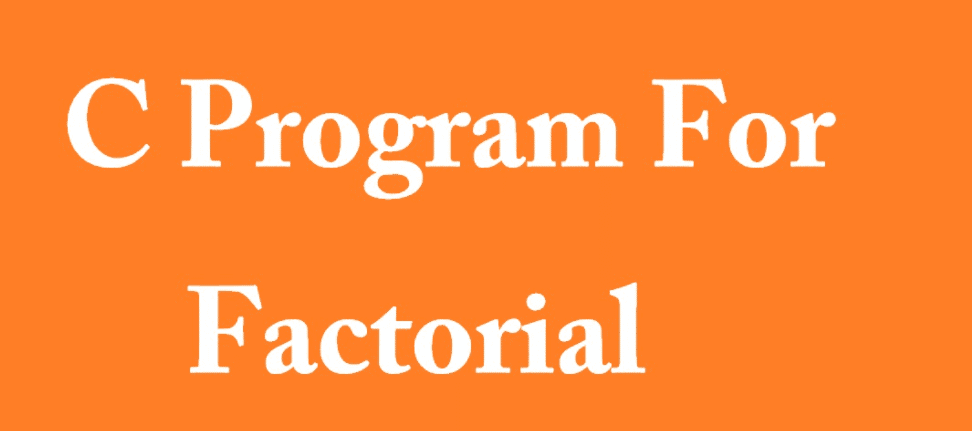## C program to find factorial of user given number – C programming guide

C program to find factorial of user given number – for beginner C program to find factorial | Let’s assume, Factorial of a positive number ‘n’ given by user factorial of n(n!) = 1 *  2 * 3 * ……. upto Nth number. Factorial of negative number is not possible. In simple word it doesn’t …